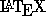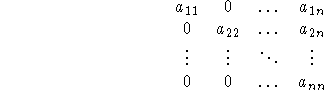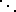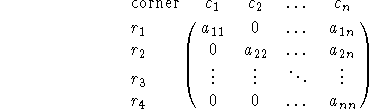Next: Case Statements Up: Commands for Math Mode Previous: Bracketing

## Matrices

You can generate a few different kinds of matrices in.

$$\begin{matrix} a_{11} & 0 & \ldots & a_{1n}\\ 0 & a_{22} & \ldots & a_{2n}\\ \vdots & \vdots & \ddots & \vdots\\ 0 & 0 &\ldots & a_{nn} \end{matrix}$$
generatesNote that the individual entries are separated by &, each matrix row ends with a \\, (carriage return) and that,

 \ldots elliptical dots\vdots vertical dots\ddots diagonal dotsare each considered as a matrix entry.

To get vertical lines or parentheses or brackets, etc. around the entries you can use the vmatrix, pmatrix, or bmatrix environments.

See theUser's Guide by Lamport for more details.

Lastly, if you want a matrix with notation on the borders, the\ construct

$$\bordermatrix{\text{corner}&c_1&c_2&\ldots &c_n\cr r_1&a_{11} & 0 & \ldots & a_{1n}\cr r_2& 0 & a_{22} & \ldots & a_{2n}\cr r_3& \vdots & \vdots & \ddots & \vdots\cr r_4& 0 & 0 &\ldots & a_{nn}}$$
givesNote that the entry corner'' appears in the corner, so if you don't want anything there you need to put a blank there explicitly.

Processed by LaTeX2html